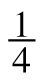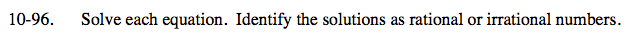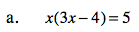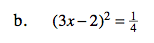### Home > CCA > Chapter 10 > Lesson 10.2.6 > Problem10-96

10-96.
1. Solve each equation. Identify the solutions as rational or irrational numbers. Homework Help ✎

1. x(3x − 4) = 5

2. (3x − 2)2 =You will need to use the Quadratic Formula.

$\textit{x}=\frac{2\pm\sqrt{19}}{3}$

IrrationalStart by taking the sqaure root of both sides.